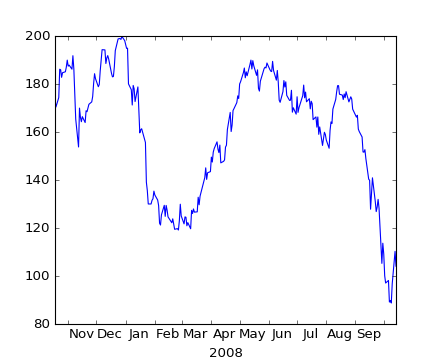### We're updating the default styles for Matplotlib 2.0

Learn what to expect in the new updatesTravis-CI:# pylab_examples example code: centered_ticklabels.py¶```# sometimes it is nice to have ticklabels centered.  mpl currently
# associates a label with a tick, and the label can be aligned
# 'center', 'left', or 'right' using the horizontal alignment property:
#
#
#   for label in ax.xaxis.get_xticklabels():
#       label.set_horizontalalignment('right')
#
#
# but this doesn't help center the label between ticks.  One solution
# is to "face it".  Use the minor ticks to place a tick centered
# between the major ticks.  Here is an example that labels the months,
# centered between the ticks

import numpy as np
import matplotlib.cbook as cbook
import matplotlib.dates as dates
import matplotlib.ticker as ticker
import matplotlib.pyplot as plt

# load some financial data; apple's stock price
fh = cbook.get_sample_data('aapl.npy.gz')
try:
# Python3 cannot load python2 .npy files with datetime(object) arrays
# unless the encoding is set to bytes. Hovever this option was
# not added until numpy 1.10 so this example will only work with
# python 2 or with numpy 1.10 and later.
except TypeError:
fh.close()
r = r[-250:]  # get the last 250 days

fig, ax = plt.subplots()

ax.xaxis.set_major_locator(dates.MonthLocator())
ax.xaxis.set_minor_locator(dates.MonthLocator(bymonthday=15))

ax.xaxis.set_major_formatter(ticker.NullFormatter())
ax.xaxis.set_minor_formatter(dates.DateFormatter('%b'))

for tick in ax.xaxis.get_minor_ticks():
tick.tick1line.set_markersize(0)
tick.tick2line.set_markersize(0)
tick.label1.set_horizontalalignment('center')

imid = len(r)/2
ax.set_xlabel(str(r.date[imid].year))
plt.show()
```

Keywords: python, matplotlib, pylab, example, codex (see Search examples)